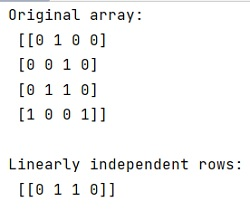# Find linearly independent rows from a matrix in Python

In this tutorial, we will learn how to find linearly independent rows from a matrix in Python? By Pranit Sharma Last updated : April 22, 2023

Given a Matrix, we have to find/identify linearly independent rows from it.

## What is median of a NumPy array?

A set of rows/vectors is said to be linearly independent if there exists no nontrivial linear combination of the rows/vectors that equals the zero vector/row in a matrix.

## How to find linearly independent rows from a matrix?

To find linearly independent rows from a matrix, you can follow the eigenvalue approach. If one eigenvalue of the matrix is zero, its corresponding eigenvector is linearly dependent. According to its documentation, it is stated that the returned eigenvalues are repeated according to their multiplicity and not necessarily ordered.

Let us understand with the help of an example,

## Python program to find linearly independent rows from a matrix

```# Import numpy
import numpy as np

# Creating an array
arr = np.array([[0, 1 ,0 ,0],
[0, 0, 1, 0],
[0, 1, 1, 0],
[1, 0, 0, 1]])

# Display original array
print("Original array:\n",arr,"\n")

# Eigen values
l, V =  np.linalg.eig(arr.T)

# Display linearly independent rows
print("Linearly independent rows:\n",arr[l == 0,:])
```

### Output